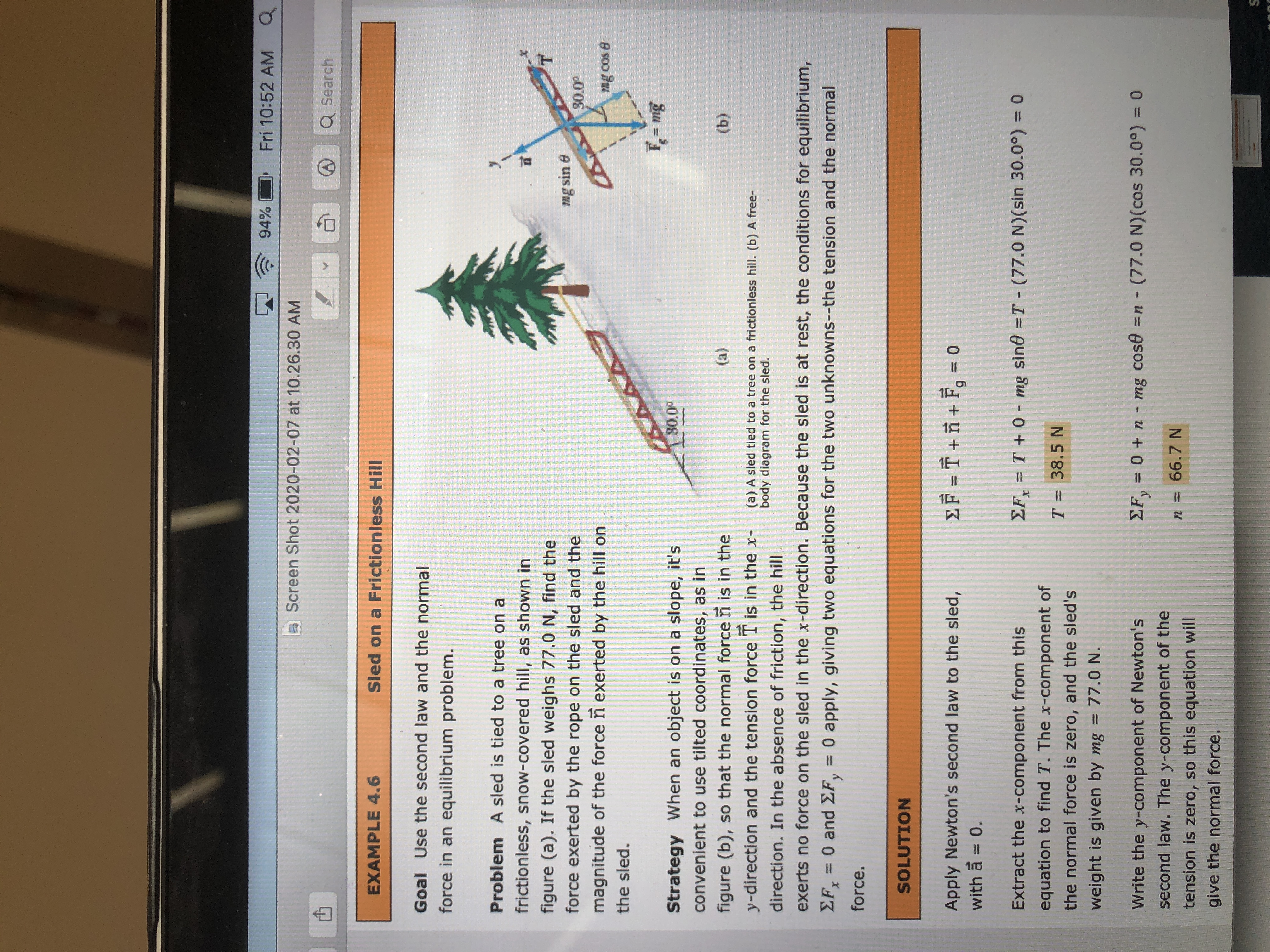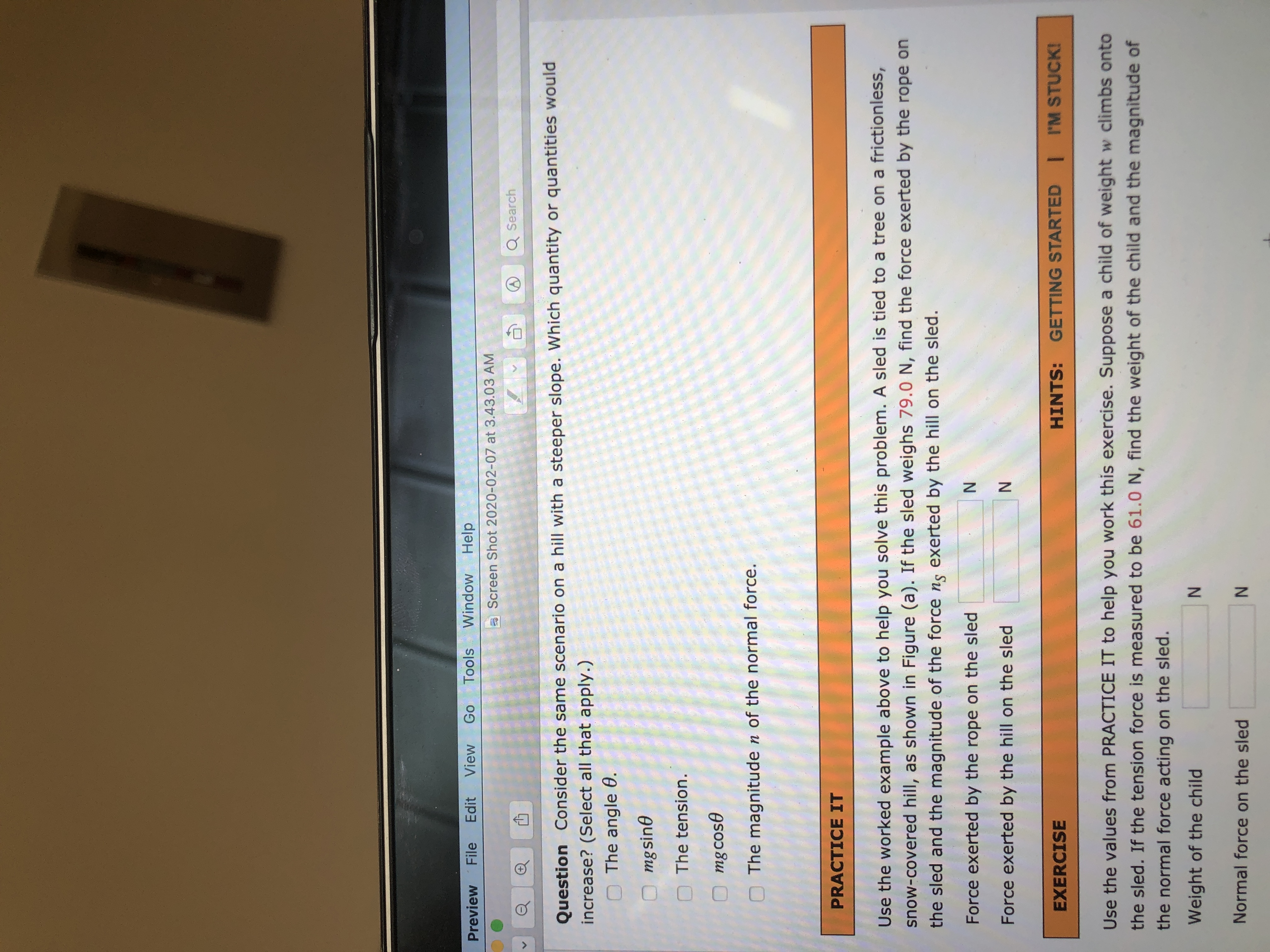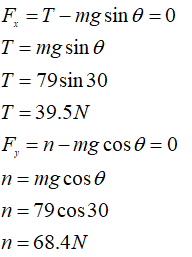# 94%Fri 10:52 AMa Screen Shot 2020-02-07 at 10.26.30 AMQ SearchEXAMPLE 4.6Sled on a Frictionless HillGoal Use the second law and the normalforce in an equilibrium problem.Problem A sled is tied to a tree on africtionless, snow-covered hill, as shown infigure (a). If the sled weighs 77.0 N, find theforce exerted by the rope on the sled and themg sin e30.00magnitude of the force ñ exerted by the hill onmg cos ethe sled.F = mig%3D80.0°Strategy When an object is on a slope, it'sconvenient to use tilted coordinates, as infigure (b), so that the normal force n is in they-direction and the tension force T is in the x-(a)(b)(a) A sled tied to a tree on a frictionless hill. (b) A free-body diagram for the sled.direction. In the absence of friction, the hillexerts no force on the sled in the x-direction. Because the sled is at rest, the conditions for equilibrium,EFx0 and Σ,0 apply, giving two equations for the two unknowns--the tension and the normal%3D%3Dforce.SOLUTIONEF = † + fñ + F¸ = 0Apply Newton's second law to the sled,with a = 0.%3DEF, = T + 0 - mg sin0 =T - (77.0 N)(sin 30.0°) = 0Extract the x-component from this%3Dequation to find T. The x-component ofT = 38.5 Nthe normal force is zero, and the sled'sweight is given by mg = 77.0 N.%3D= 0 + n - mg cos® =n - (77.0 N)(cos 30.0°) = 0Write the y-component of Newton'ssecond law. The y-component of then = 66.7 Ntension is zero, so this equation willgive the normal force. Window HelpToolsGoViewEditFilePreviewa Screen Shot 2020-02-07 at 3.43.03 AMQ SearchQuestion Consider the same scenario on a hill with a steeper slope. Which quantity or quantities wouldincrease? (Select all that apply.)O The angle 0.mgsineThe tension.mg cos0The magnitude n of the normal force.PRACTICE ITUse the worked example above to help you solve this problem. A sled is tied to a tree on a frictionless,snow-covered hill, as shown in Figure (a). If the sled weighs 79.0 N, find the force exerted by the rope onthe sled and the magnitude of the force ns exerted by the hill on the sled.Force exerted by the rope on the sledForce exerted by the hill on the sledGETTING STARTED I I'M STUCK!HINTS:EXERCISEUse the values from PRACTICE IT to help you work this exercise. Suppose a child of weight w climbs ontothe sled. If the tension force is measured to be 61.0 N, find the weight of the child and the magnitude ofthe normal force acting on the sled.Weight of the childNormal force on the sledN.

Question
1 views

this is all 1 question

much appreciated,

Thanks! ??help_outlineImage Transcriptionclose94% Fri 10:52 AM a Screen Shot 2020-02-07 at 10.26.30 AM Q Search EXAMPLE 4.6 Sled on a Frictionless Hill Goal Use the second law and the normal force in an equilibrium problem. Problem A sled is tied to a tree on a frictionless, snow-covered hill, as shown in figure (a). If the sled weighs 77.0 N, find the force exerted by the rope on the sled and the mg sin e 30.00 magnitude of the force ñ exerted by the hill on mg cos e the sled. F = mig %3D 80.0° Strategy When an object is on a slope, it's convenient to use tilted coordinates, as in figure (b), so that the normal force n is in the y-direction and the tension force T is in the x- (a) (b) (a) A sled tied to a tree on a frictionless hill. (b) A free- body diagram for the sled. direction. In the absence of friction, the hill exerts no force on the sled in the x-direction. Because the sled is at rest, the conditions for equilibrium, EFx 0 and Σ, 0 apply, giving two equations for the two unknowns--the tension and the normal %3D %3D force. SOLUTION EF = † + fñ + F¸ = 0 Apply Newton's second law to the sled, with a = 0. %3D EF, = T + 0 - mg sin0 =T - (77.0 N)(sin 30.0°) = 0 Extract the x-component from this %3D equation to find T. The x-component of T = 38.5 N the normal force is zero, and the sled's weight is given by mg = 77.0 N. %3D = 0 + n - mg cos® =n - (77.0 N)(cos 30.0°) = 0 Write the y-component of Newton's second law. The y-component of the n = 66.7 N tension is zero, so this equation will give the normal force. fullscreenhelp_outlineImage TranscriptioncloseWindow Help Tools Go View Edit File Preview a Screen Shot 2020-02-07 at 3.43.03 AM Q Search Question Consider the same scenario on a hill with a steeper slope. Which quantity or quantities would increase? (Select all that apply.) O The angle 0. mgsine The tension. mg cos0 The magnitude n of the normal force. PRACTICE IT Use the worked example above to help you solve this problem. A sled is tied to a tree on a frictionless, snow-covered hill, as shown in Figure (a). If the sled weighs 79.0 N, find the force exerted by the rope on the sled and the magnitude of the force ns exerted by the hill on the sled. Force exerted by the rope on the sled Force exerted by the hill on the sled GETTING STARTED I I'M STUCK! HINTS: EXERCISE Use the values from PRACTICE IT to help you work this exercise. Suppose a child of weight w climbs onto the sled. If the tension force is measured to be 61.0 N, find the weight of the child and the magnitude of the normal force acting on the sled. Weight of the child Normal force on the sled N. fullscreen
check_circle

Part A

If the slope is steeper it means it is more vertical and is hard to climb. So the following will increase

1. The angle θ
2. Mgsinθ because sinθ is directly proportional to θ between 0oand 90o
3. The tension because T = mgsinθ

The following will decrease

1. Mgcosθ because cosθ is inversely proportional to θ between 0oand 90o
2. The magnitude n of the normal force, because n=mgcosθ
Part B

Given

weight of the sled mg = 79 N

Angle θ = 30o

SolutionT = 39.5N

n =68.4 N

...

### Want to see the full answer?

See Solution

#### Want to see this answer and more?

Solutions are written by subject experts who are available 24/7. Questions are typically answered within 1 hour.*

See Solution
*Response times may vary by subject and question.
Tagged in

### Physics